PURE BENDING

# 1 PURE BENDING

## 1.1 Problem definition

Determine the maximum deflections and bending stress at centre of a two times simply supported beam when two downward point loads applied at the ends.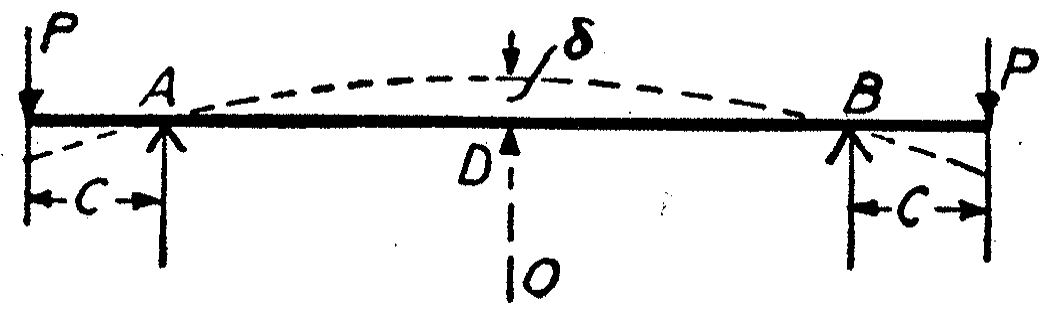Figure 1: Model by Timoshenko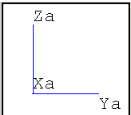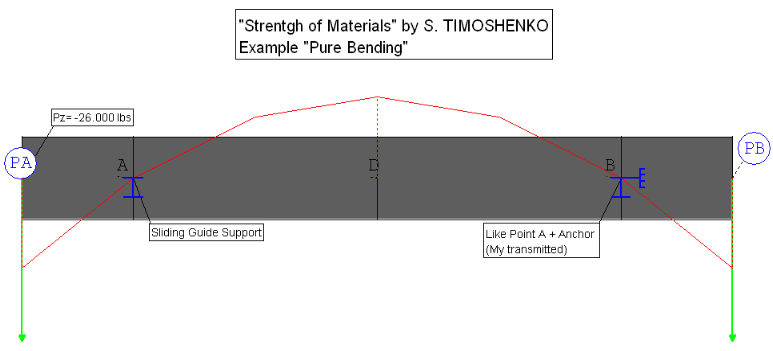Figure 2: Rohr2 Model

## 1.2 References (Timoshenko)

S. Timoshenko, Strength of Material, Part I, Elementary Theory and Problems, 3rd Edition, D. Van Nostrand Co., Inc., New York, NY, 1956, Chapter 4.23, pg. 94.

The system which consists of two boundary conditions and three unequal sections is defined by following parameters:

• Length of the stretch PA-A (â‰™C)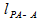• Length of the stretch PB-B (â‰™C)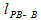• Length of the stretch A-B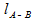• Diameter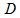• Spring load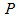• Modulus of Elasticity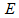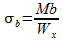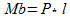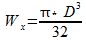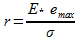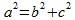Where:

 Variable Description Unit Used Value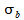Maximum stress lbs/ inchÂ² 3575,26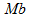Bending moment ft lbs 29250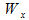Section Modulus mmÂ³ 98,175Spring load lbf 26000LengthPA;A inch 1,125LengthPB;B inch 1,125LengthAB inch 4,917Diameter inch 10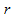Radius of curvature inch 41955,03Modulus of Elasticity lbs/ inchÂ² 30000000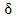Deflection inch 0,01037

Table 1: Overview of the used variables

## 1.3 Model description (ROHR2)

The whole system consists of a carbon steel beam with a ten inch diameter. It has three parts, where two of them are equal long (lPA-A; lPB-B= 13,5 inch). The third is with a length of 59 inch the longest one. At two points (PA, PB) the beams are supported. Both supports are vertical and transverse supports and at point A an addition axial stop and stop for moments of torsion is added. The system is submitted to two vertical downward force at both ends (PPA, PPB). In order to have the possibility of checking and comparing the deflections with higher precision, it is necessary to create a model where the the loads P where increased by the factor 1000. A load case is created where the gravitational acceleration isn't taken into account.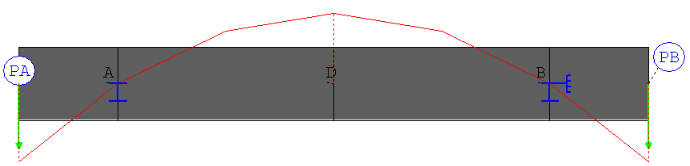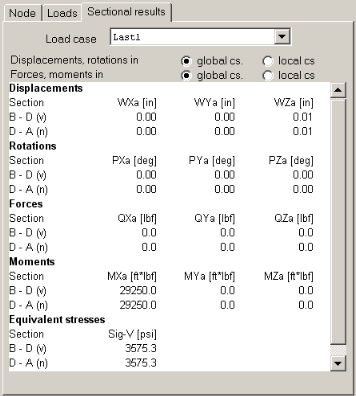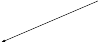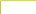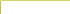Figure 3: Model with moment Informations (sectional results)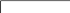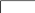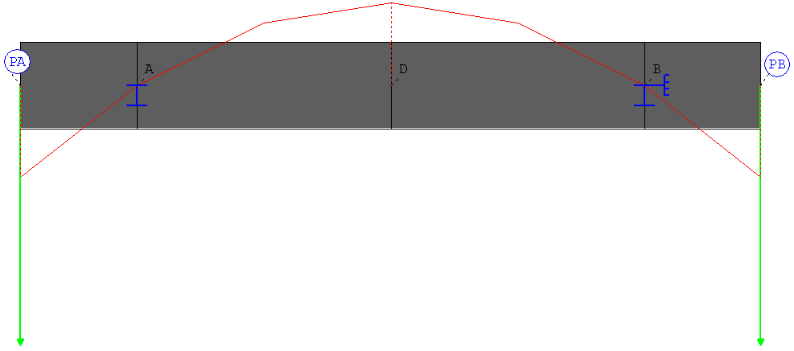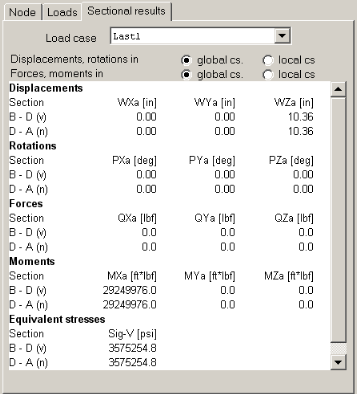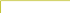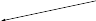Figure 4: Model with increased loads (sectional results)

## 1.4 Result comparisons

 Value Length [inch] Reference (Timoshenko) [lbs*ft] Rohr2 [lbs*ft] Difference [%]86,0 3575,26 3575,25 <0,01

Table 2: Comparison of the bending stress at point O

 Value Length [inch] Reference (Timoshenko) [inch] Rohr2 [inch] Difference [%]86,0 0,01037 0,01 <0,09

Table 3: Comparison of the max. deflection

## 1.5 Conclusion

The results perfectly match those given in the references.

## 1.6 Files

R009_inch.r2w

R009_mm.r2w

R2_stresses_9

SIGMA Ingenieurgesellschaft mbH www.rohr2.com